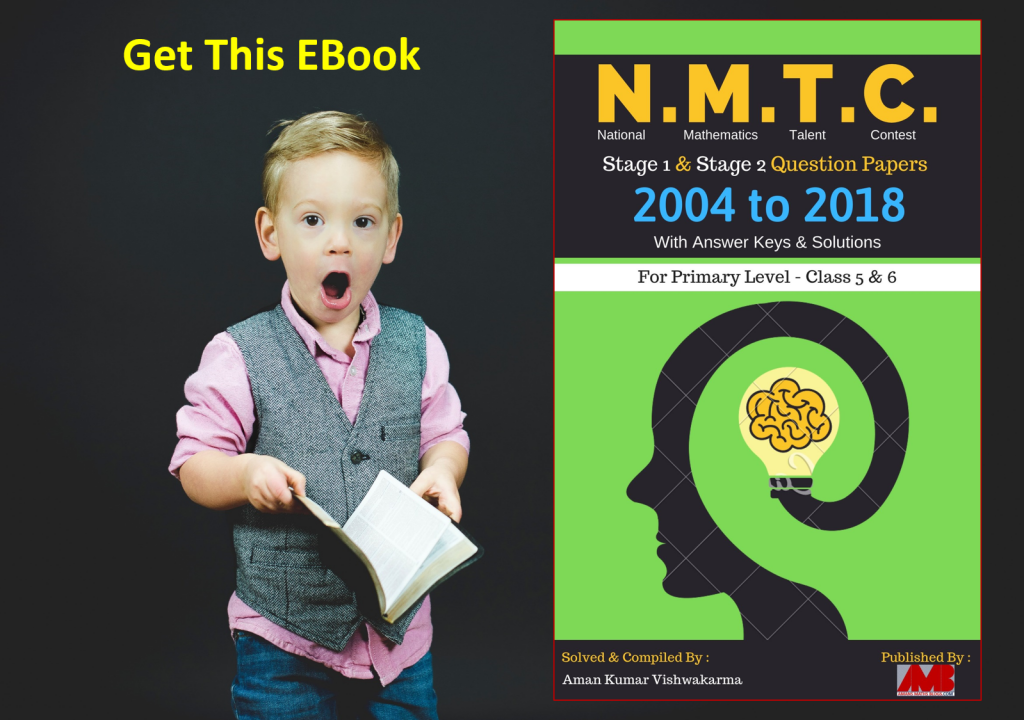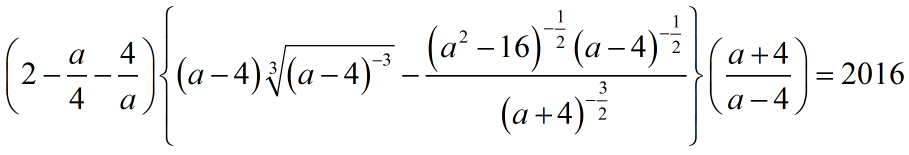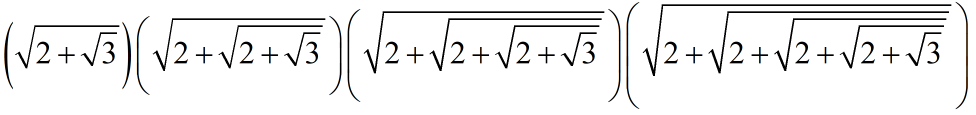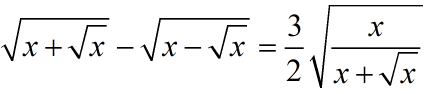Monday, January 20, 2020
Home > Latest Announcement > NMTC 2016 JUNIOR LEVEL IX & X SOLUTION in PDF & VIDEO Format

# NMTC 2016 JUNIOR LEVEL IX & X SOLUTION in PDF & VIDEO Format# NMTC 2016 Junior Level Class 9 & 10

NMTC 2016 Question Papers With Solutions Junior Level Class 9 and 10 is the exam conducted by AMTI.

## NMTC 2016 Junior Level Question Paper

NMTC 2016 Junior Level exam question paper as below.

Click Here For NMTC 2010 to 2019 Previous Year Questions Papers with Detailed Solution

To get NMTC 2004 to 2018 Questions with Solutions, Click Image Below.Click On Image To Get EBOOK

## NMTC 2016 Junior Level Solutions

Part A: Instruction:

Only One of the choices A, B, C, D is correct. For each correct response, you get 1 mark. For each incorrect response, you lose 1/2 mark.

NMTC 2016 Question Papers With Solution Junior Level Ques No 1:

The sum of the values of x and y that satisfy the equation (x + y)2(y – x) = 1 and (x + y)2(x – y) = 2 simultaneously is

Options:

A. 2

B. 3/2

C. 5/2

D. 7/2

Solution:

Read More : 129 Maths Short Tricks

NMTC 2016 Junior Level Question Papers With Solutions Ques No 2:

Ifthe value of a is

Options:

A. 4/1007

B. 3/2016

C. 4/2017

D. None of these

Solution:

NMTC 2016 Question Papers With Solution Junior Level Ques No 3:

ABCD is a square inscribed in a circle of radius of 1 unit. The tangent to the circle at C meets AB produced at P. The length of PD is

Options:

A. 2

B. 3

C.D.Solution:

NMTC 2016 Junior Level Question Papers With Solutions Ques No 4:

Quadrilateral ABCD is inscribed in a circle with radius 1 unit. AC is diameter of the circle and BD = AB. The diagonals cut at P. If PC = 2/5 then the length of CD is equal to

Options:

A. 2/3

B. 2/7

C. 1/8

D. 3/4

Solution:

NMTC 2016 Question Papers With Solution Junior Level Ques No 5:

The number of natural numbers n for which the expression (23n2 + 18n + 4)/n is also a natural number is

Options:

A. 3

B. 2

C. 1

D. 0

Solution:

NMTC 2016 Junior Level Question Papers With Solutions Ques No 6:

The cost price of 16 oranges is equal to the selling price of 12 oranges. Then there is a

Options:

A. 40% Profit

B. 20% Loss

C. 33 1/3% Profit

D. 23 1/3% Profit

Solution:

NMTC 2016 Question Papers With Solutions Junior Level Ques No 7:

The number of positive integer pairs (a, b) such that ab – 24 = 2b

Options:

A. 6

B. 7

C. 8

D. 9

Solution:

NMTC 2016 Junior Level Question Papers With Solutions Ques No 8:If A = (2 + 1)(22 + 1)(24 + 1)…(22016 + 1), then the value of (A + 1)1/2016 is

Options:

A. 4

B. 2016

C. 24032

D. 2

Solution:

NMTC 2016 Question Papers With Solutions Junior Level Ques No 9:

The sum of two numbers a, b where a < b is 1215 and their HCF is 81. The number of such pairs (a, b) is

Options:

A. 1

B. 2

C. 3

D. 4

Solution:

NMTC 2016 Junior Level Question Papers With Solutions Ques No 10:

The first Republic Day of India was celebrated on 26th January 1950. What was the day of the week on that date?

Options:

A. Tuesday

B. Wednesday

C. Thursday

D. Friday

Solution:

NMTC 2016 Question Papers With Solution Junior Level Ques No 11:

The 12 numbers a1, a2, …, a12 are in arithmetic progression. The sum of all these numbers is 354. Let P = a2 + a4 + … + a12 and Q = a1 + a3 + … + a11. If the ratio of P : Q is 32 : 27, the common ratio of the progression is

Options:

A. 2

B. 3

C. 4

D. 5

Solution:

NMTC 2016 Junior Level Question Papers With Solutions Ques No 12:

A shopkeepers marks the price of his goods at 20% higher than the original price. There is an increase in demand of the goods and he further increases the price by 20%. The total profit % is

Options:

A. 40

B. 38

C. 42

D. 44

Solution:

NMTC 2016 Question Papers With Solution Junior Level Ques No 13:

A circle passes through the vertices A and D and touches the side BC of a square. The side of the square 2 cm. The radius of the circle (in cm) is

Options:

A. 5/4

B. 4/5

C. 1

D. 5/2

Solution:

NMTC 2016 Junior Level Question Papers With Solutions Ques No 14:

There are 4 balls – one green, one red, one blue and one yellow and there are four boxes – one green, one red, one blue and one yellow. A child playing with the balls decides to put the balls in the boxes, one ball in each box. The number of ways in which the child can put the balls in the boxes such that no ball is in a box of its own color is

Options:

A. 12

B. 9

C. 24

D. 6

Solution:

NMTC 2016 Question Papers With Solution Junior Level Ques No 15:

The 5 x 5 array of dots represents trees an orchard. If you were standing at the central spot marked C, you would not be able to see of 8 of the 24 trees (shown as x). if you were standing at the center of 9 x 9 arrays of trees, how many of the 80 trees would be hidden?

Options:

A. 40

B. 32

C. 36

D. 44

Solution:

Part B: Instruction:

Write the correct answer in the space provided in the responsive sheet. For each correct response, you get 1 mark. For each incorrect response, you lose 1/4 mark.

NMTC 2016 Junior Level Question Papers With Solutions Ques No 16:

a and b are positive integers such that a2 + 2b = b2 + 2a + 5. The value of b is ______ .

Solution:

NMTC 2016 Question Papers With Solution Junior Level Ques No 17:

Fill in blank:

After full simplification, the value of the productis ____________ .

Solution:

NMTC 2016 Junior Level Question Papers With Solutions Ques No 18:

Fill in blank:

ABCD is rectangle with AD = 1 and AB = 2. DFEB is also a rectangle. The area of DFEB is ____ .

Solution:

NMTC 2016 Question Papers With Solution Junior Level Ques No 19:

Fill in blank:

The two digit number whose unit digit is exceeds the tens digit by 2 and such that the product of the number and the sum of the digits is 144 is ______ .

Solution:

NMTC 2016 Junior Level Question Papers With Solutions Ques No 20:

Fill in blank:

If x = p/q where p, q are integers having no common divisors other than 1 satisfiesthen x is ____ .

Solution:

NMTC 2016 Question Papers With Solution Junior Level Ques No 21:

Fill in blank:

AE and BF are medians drawn to the legs of a right angled triangle ABC. The numerical values of (AE2 + BF2) / AB2 is ______.

Solution:

NMTC 2016 Junior Level Question Papers With Solutions Ques No 22:

Fill in blank:

AB is a chord of a circle with center O. AB is produced to C such that BC = OA. CO is produced to E. The value of (Angle AOE) / (Angle ACE) is _______.

Solution:

NMTC 2016 Question Papers With Solution Junior Level Ques No 23:

Fill in blank:

The number of two digits numbers that are less than the sum of the squares of their digits by 11 and exceed twice the product of their digits by 5 is ______.

Solution:

NMTC 2016 Junior Level Question Papers With Solutions Ques No 24:

Fill in blank:

AB is a diameter of a circle and CD is a parallel chord. P is any point in AB. The numerical value of (PC2 + PD2) / (PA2 + PB2) is ______.

Solution:

NMTC 2016 Question Papers With Solution Junior Level Ques No 25:

Fill in blank:

In the sequence of 1, 2, 2, 4, 4, 4, 4, 8, 8, 8, 8, 8, 8, 8, 8, …, the 2016th term is 2n, then the value of n is _____.

Solution:

NMTC 2016 Junior Level Question Papers With Solutions Ques No 26:

Fill in blank:

Each root of the equation ax2 + bx + c = 0 is decreased by 1. The quadratic equation with these roots is x2 + 4x + 1 = 0. The numerical value of b + c is ______.

Solution:

NMTC 2016 Question Papers With Solution Junior Level Ques No 27:

Fill in blank:

The number of integers n such that (n + 2) / (n2 + 1) > 1/2 is _____.

Solution:

NMTC 2016 Junior Level Question Papers With Solutions Ques No 28:

Fill in blank:

P1 and P2 are two regular polygons. The number of sides of P1 and P2 respectively in the ratio 3 : 2 and the respective angles are in the ratio of 10 : 9. The number of sides of P1 and P2 is ____.

Solution:

NMTC 2016 Question Papers With Solution Junior Level Ques No 29:

Fill in blank:

In the triangle ABC, F and E are mid points of AB and AC respectively. P is any point on BC. The ratio of (Area of ABC) / (Area of FPE) is _______.

Solution:

NMTC 2016 Junior Level Question Papers With Solutions Ques No 30:

Fill in blank:

x, y, z are real and distinct numbers such that x + 1/y = y + 1/z = z + 1/x. The value of x2y2z2 is _____.

Solution:

1____ 5/2

2____ 4/1007

3____4____ 2/3

5____ 3

6____ 33 1/3%

7____ 8

8____ 4

9____ 4

10___ Thursday

11___ 5

12___ 44%

13___ 5/4

14___ 9

15___ 32

16___ 3

17___ 1

18___ 2

19___ 24

20___ 25/16

To get NMTC 2004 to 2018 Questions with Solutions, Click Image Below.Click On Image To Get EBOOK

error: Content is protected !!